Home > CC4 > Chapter A > Lesson A.1.3 > ProblemA-30

A-30.
1.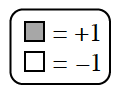Write a simplified algebraic expression for each Expression Mat below. Homework Help ✎

2.  a.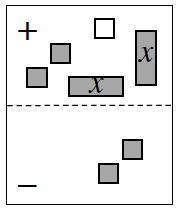b.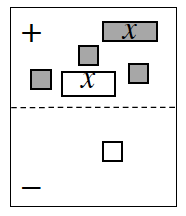c.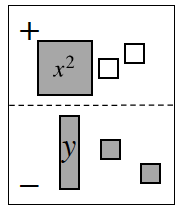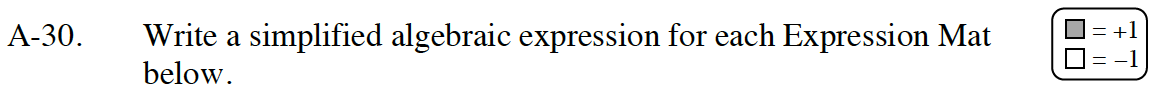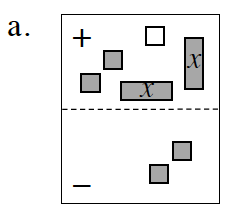Remove the zero pairs.
What is an expression for the remaining tiles?

2x − 1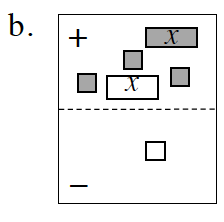On this mat, both x-tiles in the (+) region can be removed, since they make a zero pair.
Next, flip the −1 unit tile in the (-) region to the (+) region, and then simplify.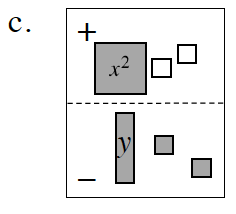Unlike in parts (a) and (b), this problem has no zero pairs.
However, here is an un-simplified expression to get you started.
x2 + (−1) + (−1) − (y + 1 + 1)
Can you simplify that expression?